You are using an outdated browser. Please upgrade your browser to improve your experience.## Taking precalculus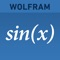# Wolfram Precalculus Course Assistant

by Wolfram Group LLC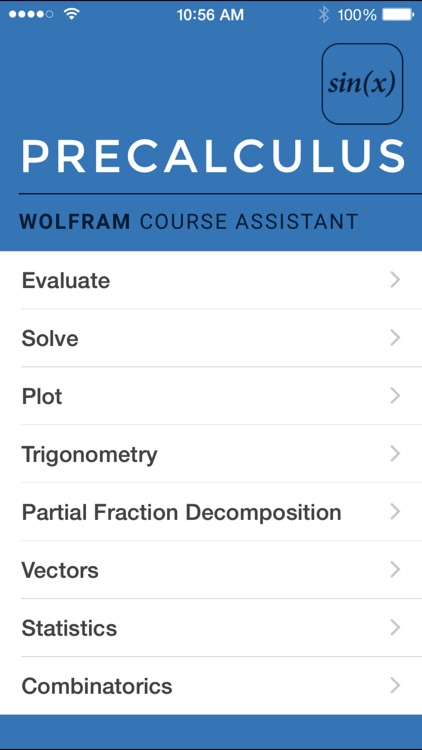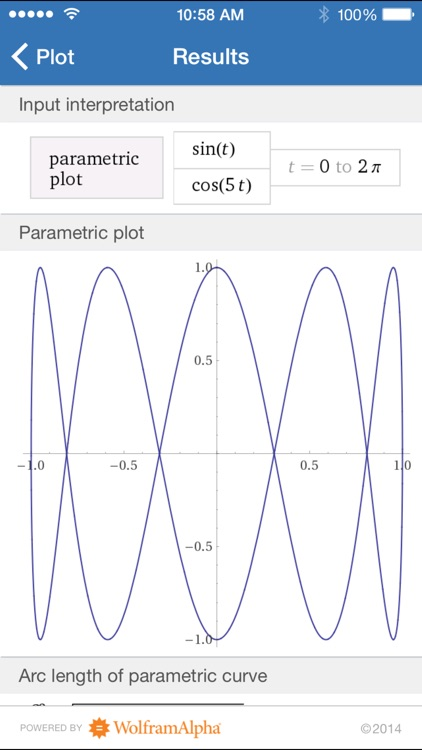### What is it about?

Taking precalculus? Then you need the Wolfram Precalculus Course Assistant. This definitive app for precalculus—from the world leader in math software—will help you work through your homework problems, ace your tests, and learn calculus concepts. Forget canned examples! The Wolfram Precalculus Course Assistant solves your specific precalculus problems on the fly, including solving equations, vector arithmetic, statistics, and much more.### App Details

Version
1.3.1
Rating
(1)
Size
14Mb
Genre
Education Reference
Last updated
May 15, 2017
Release date
May 12, 2011

### App Screenshots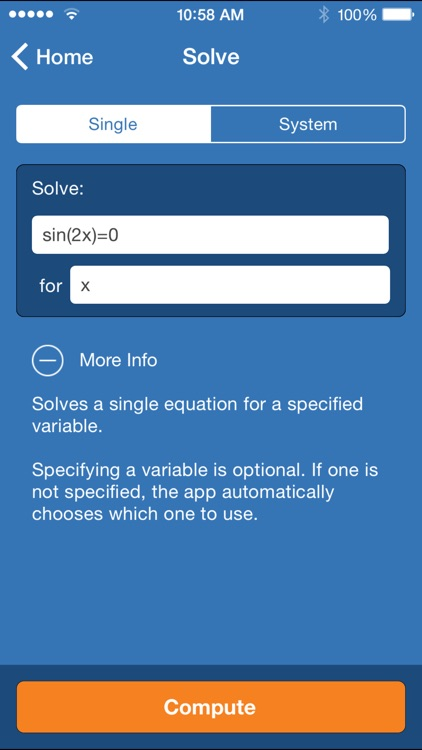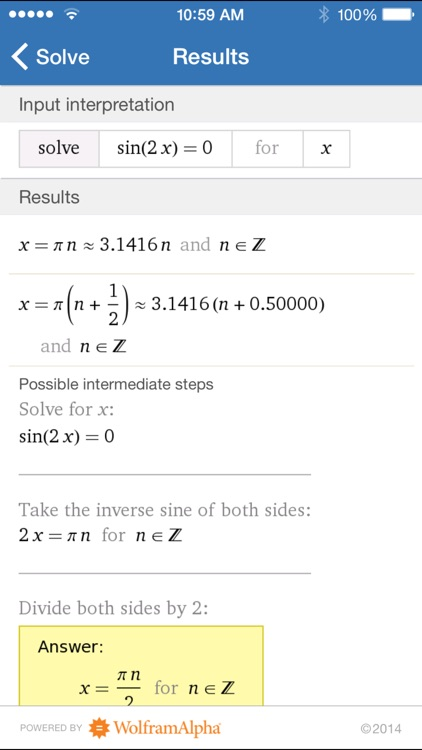### App Store Description

Taking precalculus? Then you need the Wolfram Precalculus Course Assistant. This definitive app for precalculus—from the world leader in math software—will help you work through your homework problems, ace your tests, and learn calculus concepts. Forget canned examples! The Wolfram Precalculus Course Assistant solves your specific precalculus problems on the fly, including solving equations, vector arithmetic, statistics, and much more.

This app covers the following topics applicable to precalculus and trigonometry:

- Evaluate any numeric expression or substitute a value for a variable
- Solve a single equation or a system of equations
- Plot functions on the x-y plane or draw a parametric or polar plot
- Determine the sine, cosine, and tangent of a specific angle in a right triangle
- Simplify, expand, or factor trigonometric functions
- Find the partial fraction decomposition of an expression
- Calculate the dot product, cross product, and magnitude of two vectors
- Identify the mean, median, mode, and standard deviation of a set of data
- Calculate permutations and combinations

The Wolfram Precalculus Course Assistant is powered by the Wolfram|Alpha computational knowledge engine and is created by Wolfram Research, makers of Mathematica—the world's leading software system for mathematical research and education.

The Wolfram Precalculus Course Assistant draws on the computational power of Wolfram|Alpha's supercomputers over a 3G, 4G, or Wi-Fi connection.

Disclaimer:
AppAdvice does not own this application and only provides images and links contained in the iTunes Search API, to help our users find the best apps to download. If you are the developer of this app and would like your information removed, please send a request to takedown@appadvice.com and your information will be removed.